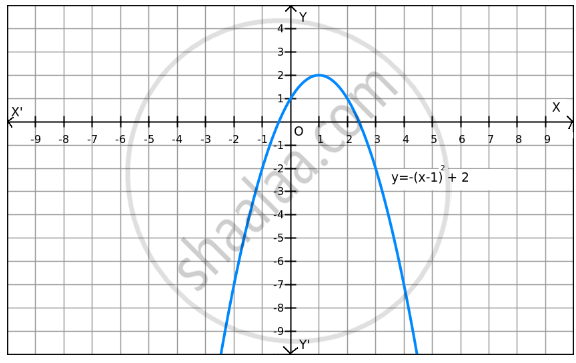PUC Karnataka Science Class 12Department of Pre-University Education, Karnataka
Share

F(X)=(X-1)2+2 on R ? - PUC Karnataka Science Class 12 - Mathematics

Question

f(x) = - (x-1)2+2 on R ?

Solution

Given: f(x) = − (x − 1)2 + 2
Now,
(x − 1)2 $\geq$ 0 for all x $\in$ R

$\Rightarrow$ f(x) = − (x − 1)2 + 2 $\leq$ 2 for all x $\in$ RThe maximum value of f(x) is attained when (x − 1) = 0.
(x − 1) = 0
⇒ x = 1
Therefore, the maximum value of f (x) = 2

Since f(x) can be reduced, the minimum value does not exist, which is evident in the graph also.

Hence, function f does not have a minimum value.

Is there an error in this question or solution?

Video TutorialsVIEW ALL 

Solution F(X)=(X-1)2+2 on R ? Concept: Graph of Maxima and Minima.
S